×#### Thank you for registering.

One of our academic counsellors will contact you within 1 working day.

Click to Chat

1800-1023-196

+91-120-4616500

CART 0

• 0

MY CART (5)

Use Coupon: CART20 and get 20% off on all online Study Material

ITEM
DETAILS
MRP
DISCOUNT
FINAL PRICE
Total Price: Rs.

There are no items in this cart.
Continue ShoppingLaw of Mass Action and Equilibrium Constant

Table of Content

Introduction of Law of Mass Action and Equilibrium Constant

Equilibrium Constant In terms of Partial Pressures

The Reaction Quotient “Q”

Significance of the Magnitude of Equilibrium Constant

Units of Equilibrium Constant

Related Resources

Introduction of Law of Mass Action and Equilibrium ConstantGuldberg and Waage established a relationship between rate of chemical reaction and the concentration of the reactants or, with their partial pressure in the form of law of mass action. According to this law,

“The rate at which a substance reacts is directly proportional to its active mass and rate of a chemical reaction is directly proportional to product of active masses of reactants each raised to a power equal to corresponding stoichiometric coefficient appearing in the balanced chemical equation”.

rate of reaction ∝ [A]a.[B]b

rate of reaction = K[A]a[B]b

where K is rate constant or velocity constant of the reaction at that temperature.

Unit of rate constant (K) = [moles/lit]1–n time–1 (where n is order of reaction.)

Note:

For unit concentration of reactants rate of the reaction is equal to rate constant or specific reaction rate.

At equilibrium the rate of both forward and backward reactions become equal and after achieving equilibria, the concentration of reactants and products remains constant as shown in figure.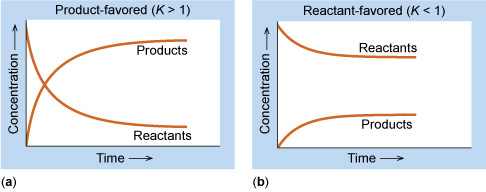Note:

Active mass is the molar concentration of the reacting substances actually participating in the reaction.

Hence,

Active mass = number of moles/volume in litres

Active mass of solid is taken as unity.

Also, Active mass of reactant (a) = Conc. × activity coefficient

i.e. a = Molarity × f                        for dilute solution f = 1

Applying Law of mass action for general reversible reaction

aA + bB  cC + dD

Rate of forward reaction  [A]a[B]b

or Rf = Kf [A]b [B]b

Similarly for backward reaction

Rb = Kb[C]c [D]d

At equilibrium Kf[A]a[B]b = Kb[C]c[D]d

The above equation is known as equilibrium equation and Kc is known as equilibrium constant.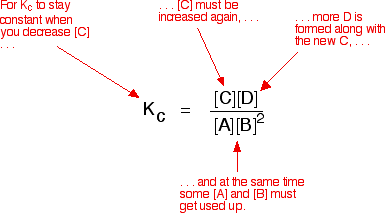The subscript ‘c’ indicates that Kc is expressed in concentrations of mol L–1
At a given temperature, the product of concentrations of the reaction products raised to the respective stoichiometric coefficient in the balanced chemical equation divided by the product of concentrations of the reactants raised to their individual stoichiometric coefficients has a constant value. This is known as the Equilibrium Law or Law of Chemical Equilibrium.

Equilibrium constant for the reverse reaction is the inverse of the equilibrium constant for the reaction in the forward direction.

Equilibrium Constant In terms of Partial Pressures (Kp)

For reactions involving gases, it is more convenient to express the equilibrium constant in terms of partial pressure.

The ideal gas equation is written as,

Where c or n/v is concentration expressed in mol/m3 (mol/L3) &  R= 0.0831 bar litre/mol K.

This indicates that at constant temperature, pressure of any gas is directly proportional to its concentration.

For reaction in equilibrium,

We can write,

Using Ideal Gas Equation we get,

PA = CART = [A] RT

PB = CBRT= [B] RT

Pc = CCRT = [C] RT

Pd = CDRT= [D] RT

Substituting the above values we get,

where ?n = (number of moles of gaseous products) – (number of moles of gaseous reactants) in the balanced chemical equation. The above given equation represents relationship between kc and kp.

Equilibrium Constant of a reaction involving Condensed Phase: The expression for the equilibrium constant of a reaction involving condensed phase (solid or liquid) and gaseous state is obtained by considering the concentrations or partial pressures of only the gaseous species. The concentration of condensed phases being constant are merged with the equilibrium constant.

a) A very large value of KC or KP signifies that the forward reaction, as written, goes to completion or very nearly so.

b) A very small numerical value of KC or KP signifies that the forward reaction, as written, does not go to any significant extent.

c) A reaction is most likely to reach a state of equilibrium in which both reactants and products are present if numerical value of KC or KP is neither very large nor very small.

d) The equilibrium constant of a forward reaction and that of its backward reaction are reciprocal of each other.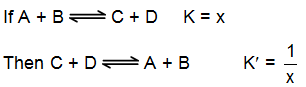e) If a chemical equation is multiplied by certain factor, its equilibrium constant must be raised to a power equal to that factor in order to obtain the equilibrium constant for the new reaction.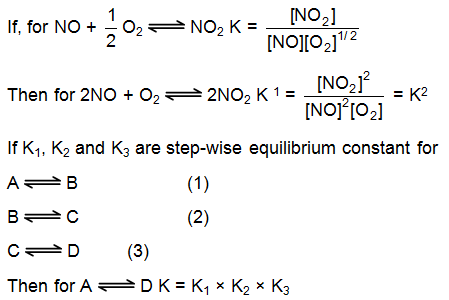The Reaction Quotient “Q”

Consider the equilibrium

PCl5 (g)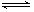PCl3(g) + Cl2 (g)

At equilibrium

When the reaction is not at equilibrium this ratio is called ‘QC’ i.e., QC is the general term used for the above given ratio at any instant of time. And at equilibrium QC becomes KC.

Similarly, PCl2 PPCl2 / PPCl2 is called QP and at equilibrium it becomes KP.

If the reaction is at equilibrium, Q = Kc

A net reaction proceeds from left to right (forward direction) if Q < KC.

A net reaction proceeds from right to left (the reverse direction) if Q >KcIllustration: For the reaction,

A(g) + B(g)2C(g) at 25°C, in a 2 litre vessel contains 1, 2, 3 moles of respectively. Predict the direction of the reaction if

(a) K­c for the reaction is 3

(b) Kc for the reaction is 6

(c) Kc for the reaction is 4.5

Solution:

A(g) + B(g)2C(g)

Reaction quotient

Q =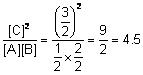(a) Q > Kc,therefore backward reaction will be followed

(b) Q > Kc  The forward reaction is followed

(c) Q = Kc The reaction is at equilibrium

Significance of the Magnitude of Equilibrium Constant

A very large value of KC or KP signifies that the forward reaction goes to completion or very nearly so.

A very small value of KC or KP signifies that the forward reaction does not occur to any significant extent.

A reaction is most likely to reach a state of equilibrium in which both reactants and products are present if the numerical value of Kc or KP is neither very large nor very small.

Units of Equilibrium Constant

As we have learned that numerical value of equilibrium constant is a function of stoichiometric coefficient used for any balanced chemical equation.

But, what will happen in the size of units of equilibrium constant when the stoichiometric coefficient of the reaction changes? (If sum of the exponent for product is not equal to reactant side sum)

So for the sake of simplicity in the units of Kp for Kc the relative molarity or pressure of reactants and products are used with respect to standard condition.

(For solution standard state 1 mole/litre and for gas standard pressure = 1 atm)

Now the resulting equilibrium constant becomes unitless by using relative molarity and pressure.

Illustration . The value of Kp for the reaction 2H2O(g) + 2Cl2(g) 4HCl(g) + O2(g) is  0.035 atm at 400oC, when the partial pressures are expressed in atmosphere. Calculate Kc for the reaction,

1/2O2(g) + 2HCl(g) —> Cl2(g) + H2O(g)

Solution:

KP = KC (RT)Δn

Δn = moles of product - moles of reactants = 5 - 4 = 1

R = 0.082 L atm/mol K,

T = 400 + 273 = 673 K

0.035 = KC (0.082 ´ 673)

KC = 6.342 ´ 10-4 mol l-1

KC for the reverse reaction would be 1/KC

KC = 1/6.342×10–4  = 1576.8 (mol l-1)-1

When a reaction is multiplied by any number n (integer or a fraction) the K'C or K'pbecomes (KC)n or (KP)n of the original reaction.

KC for 1/2 O2(g) + 2HCl(g) ——> Cl2(g) + H2O(g)

is √1576.8 = 39.7 (mol.l-1)-½

Illustration . Kp for the equilibrium, FeO(s) + CO(g) Fe(s) + CO2(g) at 1000oC is 0.4. If CO(g) at a pressure of 1 atm and excess FeO(s) are placed in a container at 1000oC, what are the pressures of CO(g) and CO2(g) when equilibrium is attained?

Solution: Assuming ideal gas behaviour, partial pressures are proportional to the no. of moles present. Since moles of CO2 formed equals moles of CO consumed, the drop in partial pressure of CO will equal the partial pressure of CO2 produced. Let the partial pressure of CO2 at equilibrium be ‘x’ atm. Then, partial pressure of CO will be (1 - x) atm.

Since  K = pCO2 / pco = x / 1 – x = 0.4  =>   x = 0.286

Hence PCO = 1-x = 0.714 atm.

Related Resources

JEE  Physical Chemistry Syllabus,

Reference books of  Chemistry

To read more, Buy study materials of Chemical Equilibrium comprising study notes, revision notes, video lectures, previous year solved questions etc. Also browse for more study materials on Chemistry here.### Course Features

• 731 Video Lectures
• Revision Notes
• Previous Year Papers
• Mind Map
• Study Planner
• NCERT Solutions
• Discussion Forum
• Test paper with Video Solution